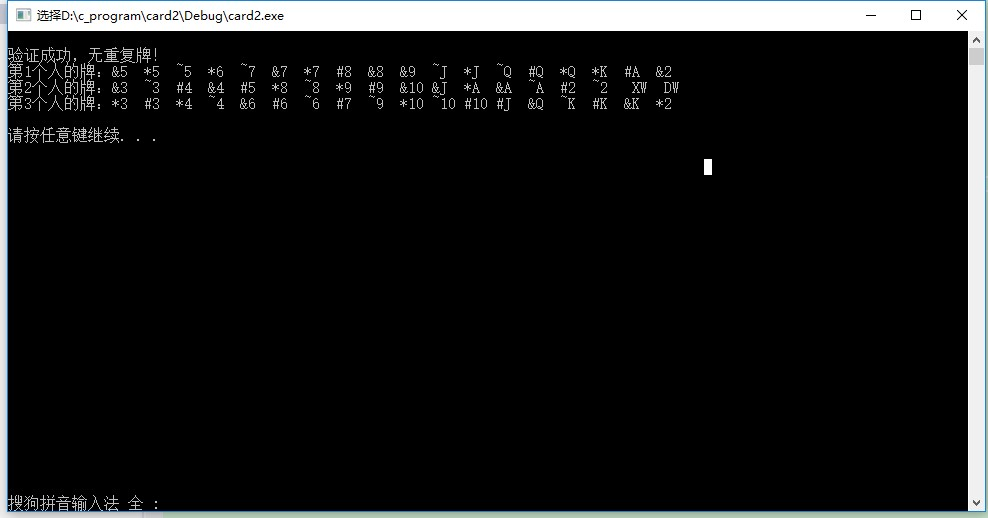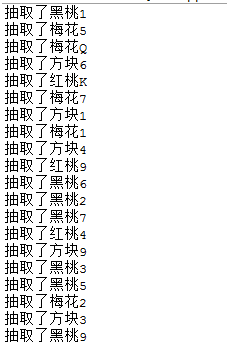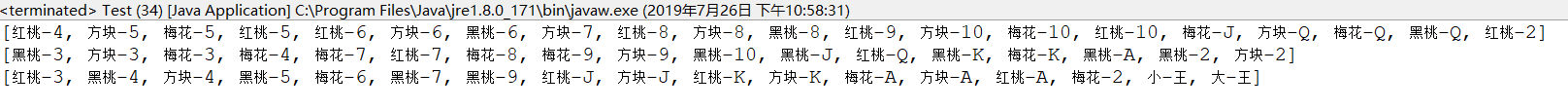• 如果要，则继续随机派给玩家一张牌，若此时所有牌的点数之和大于21，则判断玩家为输，如果不是，则玩家可以继续选择要牌与否。如果不要牌，则人类玩家阶段结束。轮到电脑。电脑阶段与玩家阶段基本相同。电脑首先自己...
• 用C语言编的随机52张给4个人，并且排序的代码
• (1) 能洗发牌； (2) 每次一手（5 张）； (3) 能评价手上的好坏； (4) 可以模拟发牌人，程序评估发牌人的，根据这...发牌人可以抓一张、两张、或三张，换掉手中不要的， 然后程序重新评估发牌人的
• 这是一副斗地主的发牌（54张加了大小王） //定义一个结构体，然后定义一个该结构体的数组Card(大写的),存储扑克（这个存储的是字符串） //定义一个int类型的card（小写的），来表示扑克，比如105，1...
前几天被问如何存储一副牌并公平发牌，就看了下，写的简单的代码如下：

<span style="font-size:12px;">
//这是一副牌斗地主的发牌（54张加了大小王）
//定义一个结构体，然后定义一个该结构体的数组Card(大写的),存储扑克牌（这个存储的是字符串）
//定义一个int类型的card（小写的），来表示扑克牌，比如105，1代表花色（红桃），05代表面值5
//再比如313，就是方块，401就是梅花A

//**********************************************************
#include<iostream>
#include<ctime>
using namespace std;
struct puke
{
charcolor;  //花色
charnumber; //牌的数值
intflag;    //3,4...J Q K A 2 XW DW
};
struct puke Card;  //加入大小王，54张，3人斗地主
int card;        //每张牌对应的整数数组
//如果按照card排序,那么牌由小到大就是:A,2,3,4,5,6,7,8,9,10,J,Q,K
//而牌由小到大排序是:3,4,5,6,7,8,9,10,J,Q,K,A,2

//*************************************************************
void _shuffleCard()   //洗牌
{
inti,j,k,temp;
for(i=0;i<4;i++)
for(j=0;j<13;j++)
card[i*13+j]=(i+1)*100+j+1;  //101 102...初始化牌的数组
card=516;//小王大王
card=517;
srand(time(NULL));   //产生随机数列的种子
for(i=0;i<54;i++)
{
k=rand()%(53-i+1)+i;   //利用随机数打乱数组
temp=card[i];
card[i]=card[k];
card[k]=temp;
}
cout<<endl;
}

//转换函数：card->Card,将牌的存储由int类型转换为char类型
void _turnCard()   //将数字转换成对应的花色的牌存入扑克数组
{
inti,temp;
for(i=0;i<54;i++)
{
temp= card[i]%100; //取牌的数值，A为1 一直K 13
Card[i].color= card[i]/100+'0';
if(Card[i].color=='1')   //为了可视化的代替红黑方梅四种不同的花色
Card[i].color='*';
if(Card[i].color=='2')
Card[i].color='&';
if(Card[i].color=='3')
Card[i].color='#';
if(Card[i].color=='4')
Card[i].color='~';
if(Card[i].color=='5')
Card[i].color='\0';
if(temp==1){
Card[i].number='A';
Card[i].number='\0';
continue;
}
if(temp==10){
Card[i].number='1';
Card[i].number='0';
continue;
}
if(temp==11){
Card[i].number='J';
Card[i].number='\0';
continue;
}
if(temp==12){
Card[i].number='Q';
Card[i].number='\0';
continue;
}
if(temp==13){
Card[i].number='K';
Card[i].number='\0';
continue;
}
if(temp==16){
Card[i].number='X';
Card[i].number='W';
continue;
}
if(temp==17){
Card[i].number='D';
Card[i].number='W';
continue;
}

Card[i].number=temp+48;
Card[i].number='\0';

}

for(i=0;i<54;i++)
{
temp= card[i]%100;
Card[i].flag= temp;
if(temp==1||temp==2)   //A 2比 3,4,,,k 大
Card[i].flag+=13;

}
//cout<<endl;

}

//*************************冒泡排序******************************
void _sortCard() //二维数组，每层排序（即每个人18张牌排序）
{
inti,j,k;
structpuke temp;
for(i=0;i<3;i++)
{
for(j=0;j<18;j++)
for(k=0;k<18-j-1;k++)
{
if(Card[i*18+k].flag>Card[i*18+k+1].flag)
{
temp= Card[i*18+k];
Card[i*18+k]= Card[i*18+k+1];
Card[i*18+k+1]= temp;
}
}

}
}

/*
void _print2()  //打印每个人的牌对应的数字
{
inti = 0;
for(;i<54;i++)
cout<<card[i]<<"";
cout<<endl;

}
*/

/* void _print3()  //检测牌重复不
{
inti = 0;
for(;i<54;i++)
cout<<Card[i].flag<<"";
cout<<endl;

}
*/

//********************************************
void _printCard()  //打印每个人的牌
{
inti,j;
for(i=0;i<3;i++){
cout<<"第"<<i+1<<"个人的牌：";
for(j=0;j<18;j++)
{
cout<<Card[i*18+j].color<<Card[i*18+j].number<<Card[i*18+j].number<<"";

}
cout<<endl;
}
cout<<endl;
}

//*****************************
void _testCard() //验牌重复不
{
inti,j,flag = 0;
for(i=0;i<54;i++)
for(j=0;j<54-i-1;j++)
{
if(i!=j)
{
if(card[i]==card[j])
flag= 1;
}
}
if(flag==0)
cout<<"验证成功，无重复牌！"<<endl;
if(flag==1)
cout<<"验证失败，存在重复牌！"<<endl;

}

int main()
{
_shuffleCard();
_turnCard();
//  _print2();
//  _print3();
_sortCard();
_testCard();
_printCard();
system("pause");
return0;
}
</span>

运行如下：展开全文C++ 随机数 排序
• 写了一个简单的扑克小程序，原理很简单，就是产生不重复的随机数
• 模拟扑克的简单算法。在这个的基础上可以多些一些代码实现自己的应用目的。 package yc415;import java.util.Random;/** * 随机发牌 * @author gong * */ public class PushCard { public static ...
模拟发扑克牌的简单算法。在这个的基础上可以多些一些代码实现自己的应用目的。  package yc415;
import java.util.Random;
/**  * 随机发牌  * @author gong  *  */  public class PushCard {
    public static  String showCard( int cardNum){
String[] hua =new String[]{"方块","梅花","红桃","黑桃"};
String[] num =new String[]{"1","2","3","4","5","6","7","8","9","10","J","Q","K"};
int huaindex=(cardNum-1)/13%4;
int numindex=(cardNum-1)%13;
return hua[huaindex] + num[numindex];
}
public static int[] gencard(int num){    // num指几副牌
int [] x = new int[ 52 * num];
for(int i=0; i<x.length; i++){
x[i]= i+1;
}
return x;
}
public static void main(String[] args) {
int [] x= gencard(1);        //举例当使用一副牌时
Random r =new Random();     //记录数组的长度
int t =0;
int length =x.length ;
for(int i=0; i<20; i++){   //控制每次抽取多少张牌
int index =r.nextInt(length);
String s =showCard(x[index]);
System.out.println("抽取了"+s);
t=x[index];
x[index]= x[length-1];
x[length-1]=t;
length--;
}

}

}展开全文算法
• 使用结构card 来描述一张牌，用随机方法来模拟人工洗牌的过程，最后将洗好的52张牌顺序分别发给四个人。 设计要求： 1）要求使用java类包中的Math.Random()方法进行随机处理。 2）要求在dos控制台窗口中显示每个人手...
• 多语言翻译器是一个小巧强大的翻译软件，该软件能翻译多种语言，并实现极速翻译，是你学习英语的好助手，让对外语不熟练的您在阅读或书写英文文章时，变得更简单更容易。
• 改程序为用JAVA语言模拟扑克发牌的过程，经测试，可以运行。
• Card类代表一张牌，其中FaceNum字段指出是牌面数字1~13，Suit字段指出的是花色，值“梅”为梅花，“方”为方块，“红”为红心，“黑”为黑桃。 Hand类代表一手牌，可以认为是一位牌手手里的牌，其中cards列表变量...
• 用C#语言编写的如何生成，取Write a console client for the Ch10CardLib library that draws five cards at one time from a shuffled Deck object. If all five cards are the same suit, then the client ...C#编程
• 利用集合模拟斗地主发牌的过程，运用TreeSet集合的自然排序特征和Map集合的键值对来匹配一张扑克java
• 主要介绍了Java模拟扑克实现生成52张扑克的方法,涉及Java数组遍历、重排及输出等相关操作技巧,需要的朋友可以参考下
• 斗地主是全国范围内的一种桌面游戏，尽管全国各种类型，但大同小异，今天我们先来实现一下斗地主中的发牌功能，为了程序的可读性以及可拓展性，此次我们使用面向对象的方法来实现此功能。 首先，斗地主要求有扑克和...
斗地主是全国范围内的一种桌面游戏，尽管全国各种类型，但大同小异，今天我们先来实现一下斗地主中的发牌功能，为了程序的可读性以及可拓展性，此次我们使用面向对象的方法来实现此功能。
首先，斗地主要求有扑克和玩家才能开始游戏，所以这里我们首先要创建一个扑克牌实体类和一个玩家实体类，具体代码如下：
首先是Poker类，含点数，花色和排序属性，大小王之后额外添加，这里我们必须实现一个Comparable接口，方便之后进行排序：
public class Poker implements Comparable<Poker>{

private String flower;
private String pointer;
private int sort;

public Poker() {

}

public Poker(String flower, String pointer, int sort) {
super();
this.flower = flower;
this.pointer = pointer;
this.sort = sort;
}

public String getFlower() {
return flower;
}

public void setFlower(String flower) {
this.flower = flower;
}

public String getPointer() {
return pointer;
}

public void setPointer(String pointer) {
this.pointer = pointer;
}

public int getSort() {
return sort;
}

public void setSort(int sort) {
this.sort = sort;
}

@Override
public String toString() {
return flower +"-" + pointer;
}

@Override
public int compareTo(Poker a) {
return this.getSort() - a.getSort();
}

}


然后就是Player类，这里我们定义属性有id，玩家名字以及玩家手牌和是否地主：
import java.util.ArrayList;

public class Player {

private int id;
private String name;
private ArrayList<Poker> pokers = new ArrayList<>();
private boolean boss;
public Player() {

}
public Player(int id, String name) {
super();
this.id = id;
this.name = name;
}
public int getId() {
return id;
}
public void setId(int id) {
this.id = id;
}
public String getName() {
return name;
}
public void setName(String name) {
this.name = name;
}
public ArrayList<Poker> getPokers() {
return pokers;
}
public void setPokers(ArrayList<Poker> pokers) {
this.pokers = pokers;
}
public boolean isBoss() {
return boss;
}
public void setBoss(boolean boss) {
this.boss = boss;
}

}

实体类建完之后，我们就要开始实现功能了，首先我们将要实现的功能简单叙述一下，首先我们要求有一副54张的完整牌，然后洗牌（此处我们用Collections里的静态shuffle方法实现），然后开始按洗好的牌发牌51张，发完随机地主，将剩余的三张牌给地主，并将三名玩家的牌均按大小显示出来。
import java.util.ArrayList;
import java.util.Arrays;
import java.util.Collections;
import java.util.List;
import java.util.Random;

public class PokerGame {

/**定义为静态以简化代码量*/
static List<Player> players;
static ArrayList<Poker> list = new ArrayList<>();
static String[] flowers = {"梅花","黑桃","方块","红桃"};
static String[] pointers = {"3","4","5","6","7","8","9","10","J","Q","K","A","2"};
int bossIndex;

static{
for(int i = 0;i < flowers.length;i++){
for(int j = 0;j < pointers.length;j++){
Poker p = new Poker(flowers[i],pointers[j],j);
}
}

players = Arrays.asList(
new Player(1,"胡灿"),
new Player(2,"戴豪杰"),
new Player(3,"马涛")
);
}

/**随机地主*/
public void pushBoss(){
/**随机一个索引，随到的为地主*/
Random r = new Random();
bossIndex = r.nextInt(players.size());
players.get(bossIndex).setBoss(true);
}

/**洗牌功能*/
public void XiPai(){
/**用Collections中的一个静态方法实现随机顺序集合*/
Collections.shuffle(list);
}

/**发牌功能*/
public void FaPai(){
/**每次按顺序给每人发一张牌直到51张牌发完*/
for(int i = 0;i < 51;i+=3){
}
/**将剩余的三张牌发给地主*/
for(int j = 51;j < 54;j++){
}
}

/**排序功能*/
public void PaiXu(){
/**用Collections的sort方法实现排序功能*/
Collections.sort(players.get(0).getPokers());
Collections.sort(players.get(1).getPokers());
Collections.sort(players.get(2).getPokers());
}

/**开始游戏*/
public void start(){
pushBoss();
XiPai();
FaPai();
PaiXu();
for(Player p:players){
System.out.println(p.getPokers());
}
}
}


下面我们来看看具体实现：
public class Test {

public static void main(String[] args) {
new PokerGame().start();
}

}

结果如下：展开全文Java
• ## java发牌程序

千次阅读 2019-11-15 20:49:11
实验5：发牌程序 题目类别: B作业 关键字: 掌握Java数组、方法的基本定义 内容要求: 编写程序，项目名和类名均为PokerGame。 实现功能： (1) 共有m幅扑克，每幅扑克不包括大王和小王共52张。 (2) 可能有n个人...
实验5：发牌程序
题目类别: B作业
关键字: 掌握Java数组、方法的基本定义
内容要求:
编写程序，项目名和类名均为PokerGame。实现功能： (1) 共有m幅扑克牌，每幅扑克牌不包括大王和小王共52张牌。 (2) 可能有n个人参与扑克游戏，2<=n<=52。 (3) 程序运行时输入扑克牌幅数m和人数n，然后所有牌分别依次分发给n个人。不能整除时，每个人的牌数可以不同，如3个人1幅牌，则第1个人18张，第2个和第3个人17张牌。 (4) 发牌完成后按花色（顺序为黑桃、红心、草花、方块）和牌面大小输出每个人得到的牌。 例如：
输入扑克牌幅数：1 输入人数：3 输出如下：
第1个人： 黑桃：K 10 5 A 红心：10 3 2 草花：K 10 8 6 3 A 方块：Q J 5 2 第2个人： … 第3个人： …
实现要求： (1) 使用数组存放发牌情况。 (2) 编写不同方法完成不同功能。
思路： 1.分牌，洗牌 2.m副牌%n个人，剩余的牌依次分给不同的人 3.平均分牌 4.排序，先输出牌多的人（牌多的人从max-1到0），后输出牌少的人（牌少的人从max-2到0）
package poker;
import java.util.Scanner;
import java.util.Arrays;

public class PokerGame {

public static void main(String[] args) {
// TODO Auto-generated method stub
Scanner input = new Scanner(System.in);

System.out.println("输入扑克牌副数:");
int m = input.nextInt();

System.out.println("输入人数:");
int n = input.nextInt();

int[][] xp = new int[m];//洗牌用
String[] hs = {"黑桃","红心","草花","方块"};//花色
String[] ph = {"A","2","3","4","5","6","7","8","9","10","J","Q","K"};//牌号
int i,j;

int max = (52*m)/n + 1;//每个人的最大牌数;
int extra = (52*m)%n;//多出来的牌数;
int[][] rpkps = new int[n][];//人数

//分配空间，拥有多一张牌数的人空间大一；
for( i = 0;i<extra;i++) {
rpkps[i] = new int[max];
}
for(j=extra;j<n;j++)
rpkps[j] = new int[max-1];

//牌初始化
for(i=0;i<m;i++)
for(j=0;j<52;j++)
xp[i][j]=j;

//洗牌
for(int k=0;k<m;k++) {
for(i=0;i<52;i++) {
int index = (int)(Math.random()*52);
int temp = xp[k][i];
xp[k][i] = xp[k][index];
xp[k][index] = temp;
}
}

//多出来的几张牌，依次发给不同的人
for(i=0;i<extra;i++) {
rpkps[i][max-1] = xp[m-1][51-extra];
}

//平均分牌
int w=0;//第几副牌
int k=0;//牌数
for(i=0;i<(52*m)/n;i++) {
for(j=0;j<n;j++) {
rpkps[j][i]=xp[w][k];
k++;
if(k==52) {
k=0;
w++;
}
}
}

for(i=0;i<n;i++)
Arrays.sort(rpkps[i]);

//先输出多的牌
for(i=0;i<extra;i++) {
//输出花色名字：
int hei=0;
int hong=1;
int cao=2;
int fang=3;

System.out.println("第"+(i+1)+"个人");
for(j=max-1;j>=0;j--) {
if(rpkps[i][j]>=39) {
if(hei == 0) {
System.out.print(hs[hei]+":");
hei = 1;
}
System.out.print(ph[rpkps[i][j]%13]+" ");
}
else if(rpkps[i][j]>=26&&rpkps[i][j]<=38) {
if(hong == 1) {
System.out.print("\n"+hs[hong]+":");
hong = 2;
}
System.out.print(ph[rpkps[i][j]%13]+" ");
}
else if(rpkps[i][j]>=13&&rpkps[i][j]<=25) {
if(cao == 2) {
System.out.print("\n"+hs[cao]+":");
cao = 3;
}
System.out.print(ph[rpkps[i][j]%13]+" ");
}
else if(rpkps[i][j]>=0&&rpkps[i][j]<=12){
if(fang == 3) {
System.out.print("\n"+hs[fang]+":");
fang = 4;
}
System.out.print(ph[rpkps[i][j]%13]+" ");
}
}
System.out.println();
}

for(i=extra;i<n;i++) {
int hei=0;
int hong=1;
int cao=2;
int fang=3;
System.out.println("第"+(i+1)+"个人");
for(j=max-2;j>=0;j--) {
if(rpkps[i][j]>=39) {
if(hei == 0) {
System.out.print(hs[hei]+":");
hei = 1;
}
System.out.print(ph[rpkps[i][j]%13]+" ");
}
else if(rpkps[i][j]>=26&&rpkps[i][j]<=38) {
if(hong == 1) {
System.out.print("\n"+hs[hong]+":");
hong = 2;
}
System.out.print(ph[rpkps[i][j]%13]+" ");
}
else if(rpkps[i][j]>=13&&rpkps[i][j]<=25) {
if(cao == 2) {
System.out.print("\n"+hs[cao]+":");
cao = 3;
}
System.out.print(ph[rpkps[i][j]%13]+" ");
}
else if(rpkps[i][j]>=0&&rpkps[i][j]<=12){
if(fang == 3) {
System.out.print("\n"+hs[fang]+":");
fang = 4;
}
System.out.print(ph[rpkps[i][j]%13]+" ");
}
}
System.out.println();
}
input.close();
}
}


展开全文java
• 分析：将要按照花色进行编号。 梅花：0-12，方片：13-15，红桃：26-38，黑桃：39-51。 然后把所有的编号存储在一个列表中。 定义洗函数，通过多次随机产生两个位置索引并交换两个位置的，达到洗目的。...python
• ## 洗牌和发牌算法

千次阅读 2015-08-21 16:42:09
而无论采用什么算法，其本质都是使得每个人获得每一张牌的概率都相同。这样的话，就有且只有两种思路，一种是洗牌，顺序牌，一种是不洗牌，随机牌。其中后一种思路又可以分为随机抽牌顺序算法
• 纯CSS实现扑克效果，太牛了吧？开始我还以为是一张图片呢，后来发现代码里没有调用任何图片，顿时有点诧异，CSS果然很牛，或是作者水平比较牛？
• Card类代表一张牌，其中FaceNum字段指出是牌面数字1~13，Suit字段指出的是花色，值“梅”为梅花，“方”为方块，“红”为红心，“黑”为黑桃。 Hand类代表一手牌，可以认为是一位牌手手里的牌，其中cards列表变量...python 编程语言
• &lt;!DOCTYPE html&gt; &lt;html&gt; &lt;head&gt; &lt;meta charset="UTF-8"&gt; &lt;... //数值从大到小,方便后面排序 //大 小 王
• key 对应的是编号 , value 是 牌的花色(红方梅黑)+ 具体的一张牌 ,比如 黑桃2 用2个数组 存放 花色+ 13张牌 将牌的信息 存入到 list和map 牌了… (需要打乱顺序) 看一下 自己是什么牌 (看牌) 由于代码...java
• 一张扑克可用结构类型描述，一副扑克的52张则是一个结构数组。 1、试编写洗函数和供4人玩牌的发牌函数。 2、统计出现同花顺的概率 提示：模拟发牌100000次（这个次数可用#define设置），程序最后统计如下...C语言 同花顺
• ## java实现斗地主发牌

千次阅读 2019-09-05 08:55:27
以及同一轮中，当一张牌已经被发给第一个人时，再牌给下一个人这张牌不可以再出现。 设计 首先考虑一个斗地主游戏中有什么对象，我认为斗地主中应该会有玩家，还有牌，另外还需要一个牌的人，我比较懒，觉得玩家...java
• # 一张牌 def __init__(self, suite, face): self._suite = suite self._face = face @property def suite(self): return self.suite @property def face(self): return self._face def __str__(self)...扑克游戏 python python游戏
• 4名牌手打牌，计算机随机将52张牌...Card类代表一张牌，其中FaceNum字段指的是牌面数字1-13，Suit字段指的是花色，Rank指的是牌的大小 (1)Card构造函数根据参数初始化封装的成员变量，实现盘面大小和花色的初始化...
• ## 斗地主之洗牌发牌----Java篇

多人点赞 热门讨论 2021-06-11 15:40:09
实现斗地主过程中的洗发牌和看。 并且确保每一位玩家手上拿到的是随机并且按照大小排序好的 思路 创建一个牌盒，也就是定义一个集合对象，用ArrayList集合实现 往牌盒里面装，也就是把打散，用...
• 发牌开始后，随机从未发牌堆中抽取一张，并发给第一个个人，然后抽取第二张，发给第二个人，重复这个流程直到52张牌发完为止。玩牌的人数为固定4人。 发牌完毕后，请将每个人拿到的按照红桃、黑桃、方块和梅花...
• Card类代表一张牌，其中FaceNum字段指出是牌面数字1~13，Suit字段指出的是花色，值“梅”为梅花，“方”为方块，“红”为红心，“黑”为黑桃。 Hand类代表一手牌，可以认为是一位牌手手里的牌，其中cards列表变量...python
• 昨天看了一个痘印，一个使用js实现的斗地主发牌。当时来了兴趣，就想着用JAVA实现一个发牌。虽然没有js配合前端页面那么炫酷。但也是整体实现了，全程自己的思路。就当是锻炼一下把。 说一下大概思路： 扑克...
• 发牌程序 题目类别: B作业 关键字: 掌握Java数组、方法的基本定义 内容要求: 编写程序，项目名和类名均为PokerGame。 实现功能： (1) 共有m幅扑克，每幅扑克不包括大王和小王共52张。 (2) 可能有n个人参与......